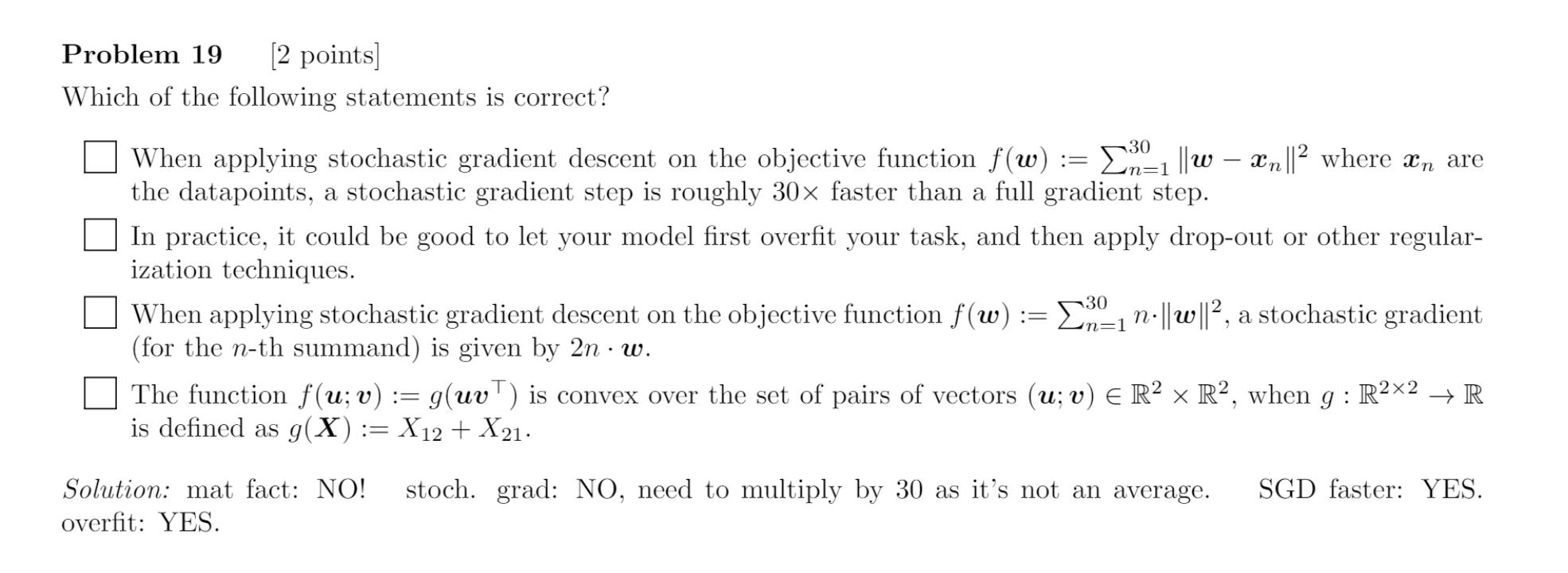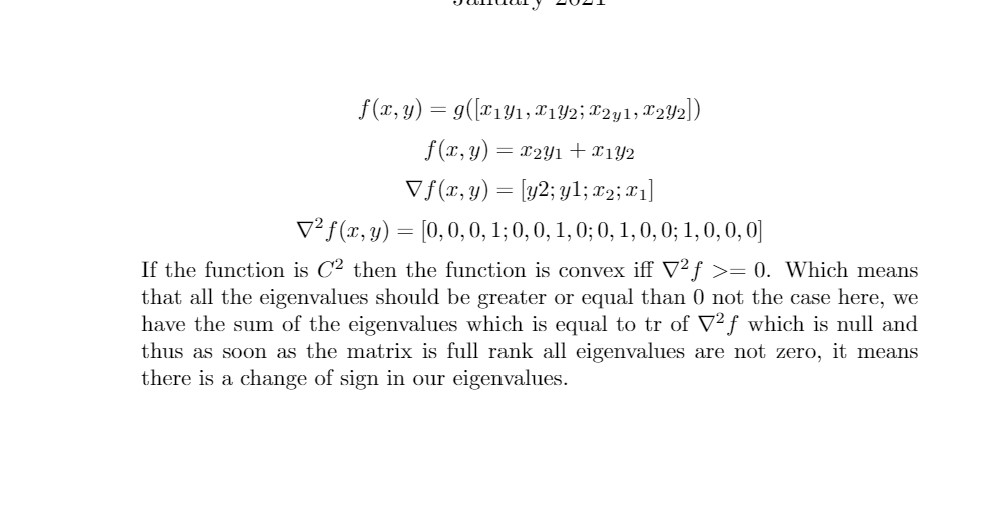### Problem19 Convex

Could someone explain to me the fourth choice. Why is it not convex?I am also a bit confused about the 'overfit' and the second SGD. In my opinion :

• you need to apply regularization + dropout during the training not after as suggested in the mcq
• what do they mean by 'you need to multiply by 30 as it's not an average'

And to answer to your question I think that it's simply because the function is not continuous over $$R^{2\times 2}$$.. Not sure thoughI actually didn't understand the motivation for the last answer.
Moreover I don't understand why the third answer is wrong: since it says the gradient for the n-th summand is $$2nw$$, I thought it was right, because then the gradient for the whole sum of 30 elements would be the sum of each of the n-th summand gradient. Is this wrong?

Stoch gradient considers loss function as $$1/N*sum(...)$$ and takes the inner of the sum when there is the 1/N in front of it. Here that term isnt there, so you have to rewrite f as $$1/30 sum(30 ... )$$. Which is why you get $$30*2*n*w$$

You are right, and I would just add that loss function L has to be an average since otherwise SGD and GD won't give the same results in expectation.

@jeangillain said:I don't find it clear how you know that you have negative eigenvalues by looking at this matrix.
Could you please share more details :)
As in the link with trace etc

Thanks a lot

Hello, I also don't see why the eigenvalues are negative. Any help will be useful.
Thanks

1. The hessian is full rank. This can be seen easily because if we re-arrenge the columns we get the identity matrix.
Since the hessian is full rank we know that the eigenvalues are NOT zero.

2. The sum of the eigenvalues is equal to the trace of the hessian. The trace of the hessian is 0. For the sum to be zero (since we know that the eigenvalues are not zero), then some eigenvalues must be positive and others negative.

3. Since some eigenvalues are negative and others positive, thus it is not positive semi-definite, therefore it is not convex.

A 2x2 matrix is positive definite if and only if the diagonal terms are positive and the determinant is positive.

From Wikipedia:
The Hessian matrix of a convex function is positive semi-definite.
The second-derivative test for functions of one and two variables is simple. In one variable, the Hessian contains just one second derivative; if it is positive, then x is a local minimum, and if it is negative, then x is a local maximum; if it is zero, then the test is inconclusive. In two variables, the determinant can be used, because the determinant is the product of the eigenvalues. If it is positive, then the eigenvalues are both positive, or both negative. If it is negative, then the two eigenvalues have different signs. If it is zero, then the second-derivative test is inconclusive.

https://en.wikipedia.org/wiki/Convex_function

Page 1 of 1